\$B:#>,\$N0lGU(B

\$BKhF|!":#>,\$N0lGU\$r>e\$2\$F\$\$\$^\$9!#(B
\$B:#>,0{\$`\$b\$N\$K:\$\$C\$?\$i!";29M\$K\$7\$F\$_\$F\$/\$@\$5\$\$!#(B

2021\$BG/(B10\$B7n(B

 1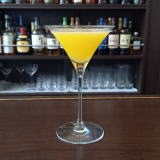(I5Z]<^%8^[0L^(B 2(IK^/8^K_0D\$B=@F;(I4C^(<.](B 3(IK^/8^K_0D\$B>-4}(I4C^(<.](B 4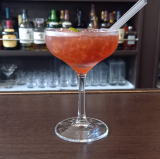(I=[0%WQ%LW/M_(B 5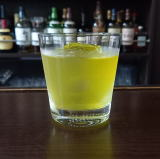(IZD[(B 6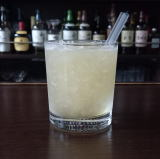(IR0?]%C^(8?](B 7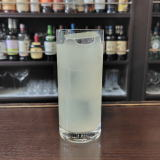(IC^(@%=L_S0F(B 8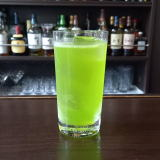(IR[]%=X]8^(B 9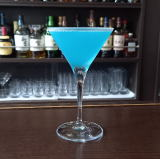(IM_6^;=(B 10 \$B5Y\$_(B 11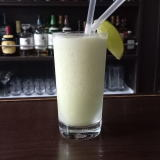(I8^X0]%12=^(B 12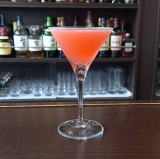(I=60Z/D%ZC^((B 13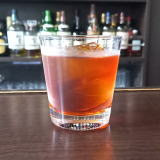(ISX]N0Y(B 14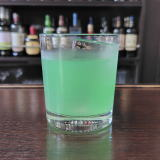(IOXL^%@^];0(B 15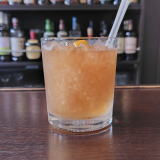(I<^]<^,0%P=D(B 16(IN0>=^%H/8(B 17 \$B5Y\$_(B 18(IM_<*%[\2TY(B 19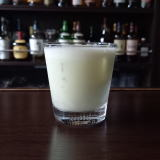(I8^X0]%J^0D^(B 20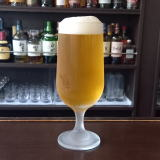(I;L^OXI(B 21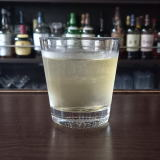(IY<1]%H2Y(B 22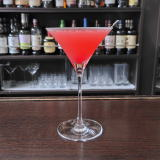(IJ^6YC^(10I(B 23(IL_XR0W(B 24 \$B5Y\$_(B 25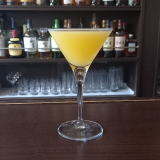(IJ^EE26(B 26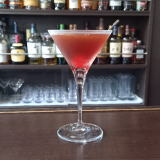(IJ_E<*(B 27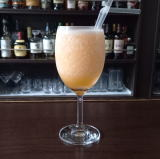(I1L_X:/D%ZC^(0(B 28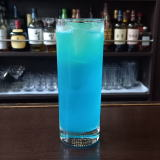(IN_1]%L^Y0(B 29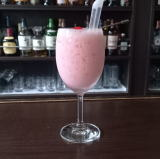(I4Y%LWP]:^(B 30(I1[O%DW/L_(B 31 \$B5Y\$_(B